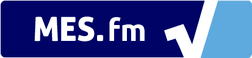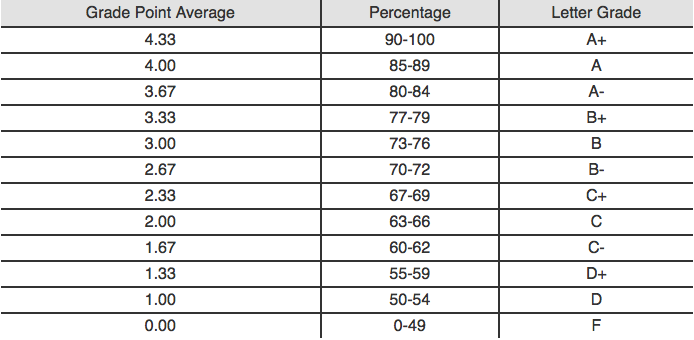We notice that you are using AdBlock. Please whitelist GPA Calculator to help keep this site up and running! :)# Grade Point Average: Introduction and History to GPA

The percentage that a student receives in a course is useful in measuring his understanding of the topics studied; however, when looking at the student’s entire semester, using an average percentage would pose serious issues.

For example, one course may have been worth just one credit and yet the student received 100% which would bump up his semester average. This is where Grade Point Average (GPA) is very useful. Not only does it take into effect individual course marks, it uses the weight of each course in the calculation to have a better indication of the students’ academic status.

GPA is the total amount of grade points that a student earns divided by how many credits it took to earn them. In order to earn grade points, a student must score within the percentage ranges in the chart below. Scoring an 83% in math for example would deem the student a GPA of 3.67 in that course. In order to find the total grade points the student earned from that course, you would simply multiply the GPA by the amount of credits the course was worth.## GPA = Total Grade Points Earned / Total Credits Taken

For example: if a student receives 93% in a course that was worth 2 credit and 71% in a course that was worth 4 credits. What GPA would this student have obtained between these two courses?

Answer: What you would do is simply find the percentage range that it lies between and what GPA is associated with it. In this case the 93% would be associated with a 4.33 GPA. Since the course is worth 2 credits you would multiple it by two and get the grade points earned which would be 8.66. Now what you would do is find what GPA the 71% is associated with. It happens to be 2.67 GPA. Now what you would do is multiply that by the amount of credits, in this case 4.

This would give a grade points earned of 10.68. Now you would add up the 10.68 and 8.66 to get your total grade points earned which would add up to be 19.33. We now have the numerator of the GPA equation above all figured out. All we have to do now is divide it by the total number of credits that the courses possess (total credits taken). After the calculation, you would have a final GPA of approximately 3.22.